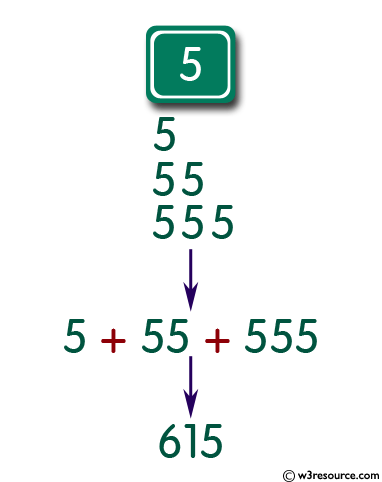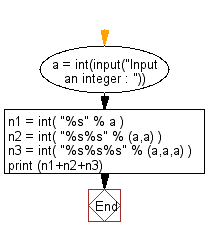﻿ Python: Input an integer (n) and computes the value of n+nn+nnn - w3resource# Python: Input an integer (n) and computes the value of n+nn+nnn

## Python Basic: Exercise-10 with Solution

Write a Python program that accepts an integer (n) and computes the value of n+nn+nnn.

Sample value of n is 5

Python int(x, base=10):

The function returns an integer object constructed from a number or string x, or return 0 if no arguments are given. If x is a number, return x.__int__(). For floating point numbers, this truncates towards zero.

• If x is not a number or if base is given, then x must be a string, bytes, or bytearray instance representing an integer literal in radix base
• The literal can be preceded by + or - (with no space in between) and surrounded by whitespace
• A base-n literal consists of the digits 0 to n-1, with a to z (or A to Z) having values 10 to 35. The default base is 10.
• The allowed values are 0 and 2-36. Base-2, -8, and -16 literals can be optionally prefixed with 0b/0B, 0o/0O, or 0x/0X, as with integer literals in code.

Pictorial Presentation:Sample Solution :-

Python Code:

``````a = int(input("Input an integer : "))
n1 = int( "%s" % a )
n2 = int( "%s%s" % (a,a) )
n3 = int( "%s%s%s" % (a,a,a) )
print (n1+n2+n3)
``````

Sample Output:

```615
```

Flowchart:## Visualize Python code execution:

The following tool visualize what the computer is doing step-by-step as it executes the said program:

Python Code Editor:

Have another way to solve this solution? Contribute your code (and comments) through Disqus.

What is the difficulty level of this exercise?

Test your Python skills with w3resource's quiz

﻿

## Python: Tips of the Day

Unpack variables from iterable:

```# One can unpack all iterables (tuples, list etc)
>>> a, b, c = 1, 2, 3
>>> a, b, c
(1, 2, 3)

>>> a, b, c = [1, 2, 3]
>>> a, b, c
(1, 2, 3)
```